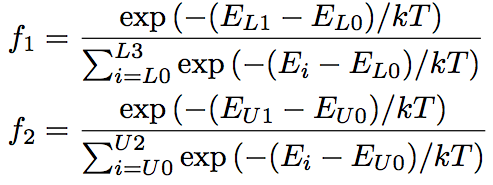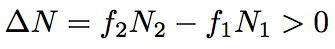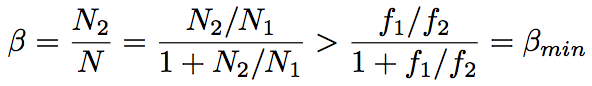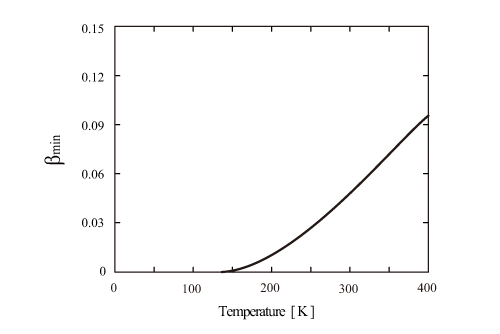Energy difference among the stark levels in Yb silica glass is around 1000 cm-1 for both the ground and excited state. The laser lower state can be thermally excited at room temperature. Therefore, the Yb silica glass laser is relatively hard to achieve the inverse distribution compared with a four-state laser. Because a lifetime of ground and excited states are as short as picosecond order, we consider a thermal equilibrium between assistant levels for most of the laser operations, and then an electronic distribution follows a Boltzmann distribution. Now, let us think of a temperature dependency of energy distribution in Yb silica glass. f1, a rate of Yb3+ in the level L1 to the total number of Yb3+ in the ground state, 2F7/2 (N1), and f2, a rate of Yb3+ in the level U0 to the total number of Yb3+ in the excited state, 2F5/2 (N2), are expressed by the following equations,where k is a Boltzmann constant, 1.38 x 10-23 [J/K], T is temperature, Ei is energy at level i, respectively. The condition for the inverse distribution is expressed as follows.When we have β, a rate of N2 to the total number of Yb3+ (N), the minimal pumping rate necessary for achieving the inverse distribution is shown as follows.βmin = 0 for a four-states system, βmin = 0.5 for a three-states system. Figure represents βmin of Yb silica glass as a function of temperature. In order to obtain the inverse distribution at room temperature, approximately 5 % of Yb3+ must be excited to the upper state. As the temperature decreases down to 100 K or lower, the minimal pumping efficiency decreases down to 0.02 % or less, then Yb silica glass laser can be seen as a four-states system.Fig. Minimum pumping rate necessary for inverse population